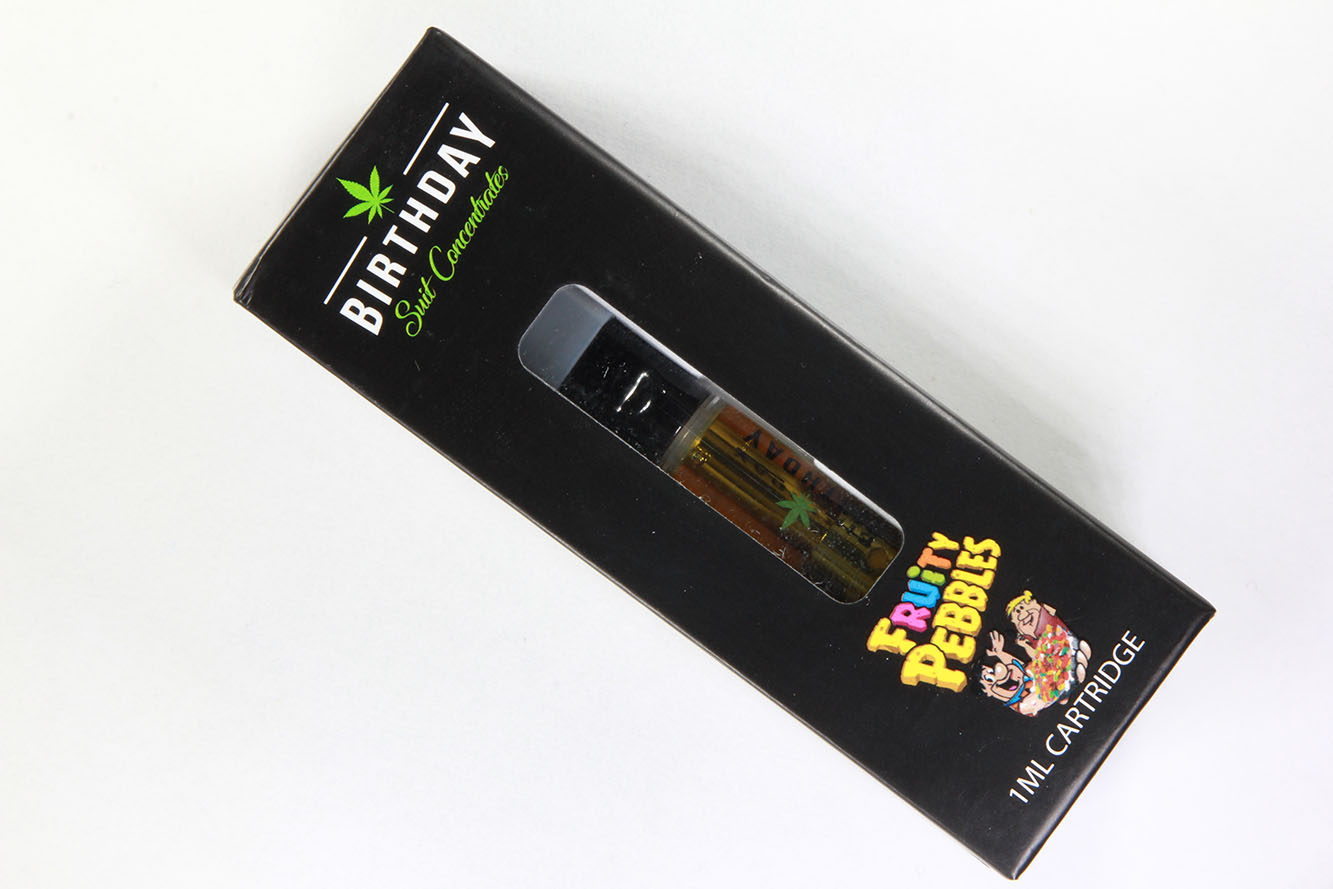83.33%

Total Cannabinoids

-

Total Terpenes
BATCH # N/A - Tested 12/12/2021 for Potency

POTENCY PROFILES

Cannabinoid Profile

83.33%
Total Cannabinoids
74.4%
Max THC
1.03%
Max CBD

Cannabinoids are quantified using high-performance liquid chromatography.

Total Cannabinoids is the sum of all detected cannabinoids.

Max THC THCa is converted to THC by heat. Max THC is the maximum amount of THC that can be derived from this product. Use the following formula to find the Max THC: Max THC = THC + (THCa * 0.877)

Max CBD CBDa is converted to CBD by heat. Max CBD is the maximum amount of CBD that can be derived from this product. Use the following formula to find the Max CBD: Max CBD = CBD + (CBDa * 0.877)

Other* This represents materials other than cannabinoids in the product.

To determine how much Fruity Pebbles to consume to achieve your desired dose, use the:

mg
﻿﻿